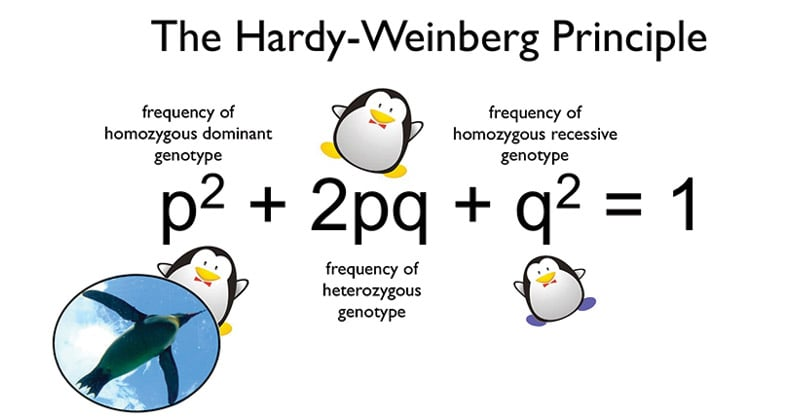# Hardy-Weinberg Equilibrium

• The Hardy–Weinberg principle, also known as the Hardy–Weinberg equilibrium, model, theorem, or law explains that allele and genotype frequencies in a population will remain constant from generation to generation in the absence of other evolutionary influences.
• The law was proposed by a British mathematician Hardy and a German physician Weinberg (1908) independently.
• Both the ideas together is called as the Hardy-Weinberg law equilibrium after their names.
• It states that both gene frequencies and genotype frequencies will remain constant from generation to generation in an infinitely large interbreeding population in which mating is at random and no selection, migration or mutation occurs.Assuming certain conditions, the Hardy-Weinberg equilibrium thus proposes that allelic frequency of a population will stay stable over time.

## Conditions of the Hardy-Weinberg population are:

• The population must be large.
• Mating must be random, with no selection for certain genotypes.
• There must be no emigration or immigration.
• There may be no mutations.
• There may be no incestuous mating.

## THE EQUATIONS

• Suppose in a population of plant species, a gene has two allele, ‘A’ n ‘a’ having frequency ‘p’ n ‘q’ respectively.
• The frequencies of their genotypes AA, 2Aa and aa are p,2pq and q.
• According to this law, the frequency of p and q in a population remains constant generation after generation unless there’s any factor’s influence.

Mathematically,

If there is a gene with two alleles, A and a.

p + q = 1                   (Eq. 1)

• p = frequency of A allele
• q = frequency of a allele

p2 + 2pq + q2 = 1     (Eq. 2)

• p2 = frequency of AA genotype
• 2pq = frequency of Aa genotype
• q2 = frequency of aa genotype

## Inference

As shown by Hardy and Weinberg, alleles segregating in a population tend to establish equilibrium with reference to each other. Thus, if two alleles should occur in equal proportion in a large, isolated breeding population and neither had a selective or mutational advantage over the other, they would be expected to remain in equal proportion generation after generation.

## Applications

The Hardy–Weinberg principle may be applied in two ways, either a population is assumed to be in Hardy–Weinberg proportions, in which the genotype frequencies can be calculated, or if the genotype frequencies of all three genotypes are known, they can be tested for deviations that are statistically significant.

## SAMPLE PROBLEM INVOLVING HARDY-WEINBERG EQUILIBRIUM

QUESTION

Sickle cell anemia, an autosomal recessive disease, occurs in 1 of every 400 African-American births. What is the frequency of carriers of the sickle cell gene in this population?

Carriers (or heterozygotes) are represented by 2pq, so we need to solve for p and q.

We are told that q 2 is equal to 1/400 or 0.0025.

Thus, q = 0.05

We know that p + q = 1, so p = 1 – q. In this case,

p = 1 – 0.05 = 0.95.

We then solve for 2pq, which is equal to 2(0.95) (0.05) or 0.095.

So 95 of every 1000 African- Americans carry the sickle cell gene.

## References